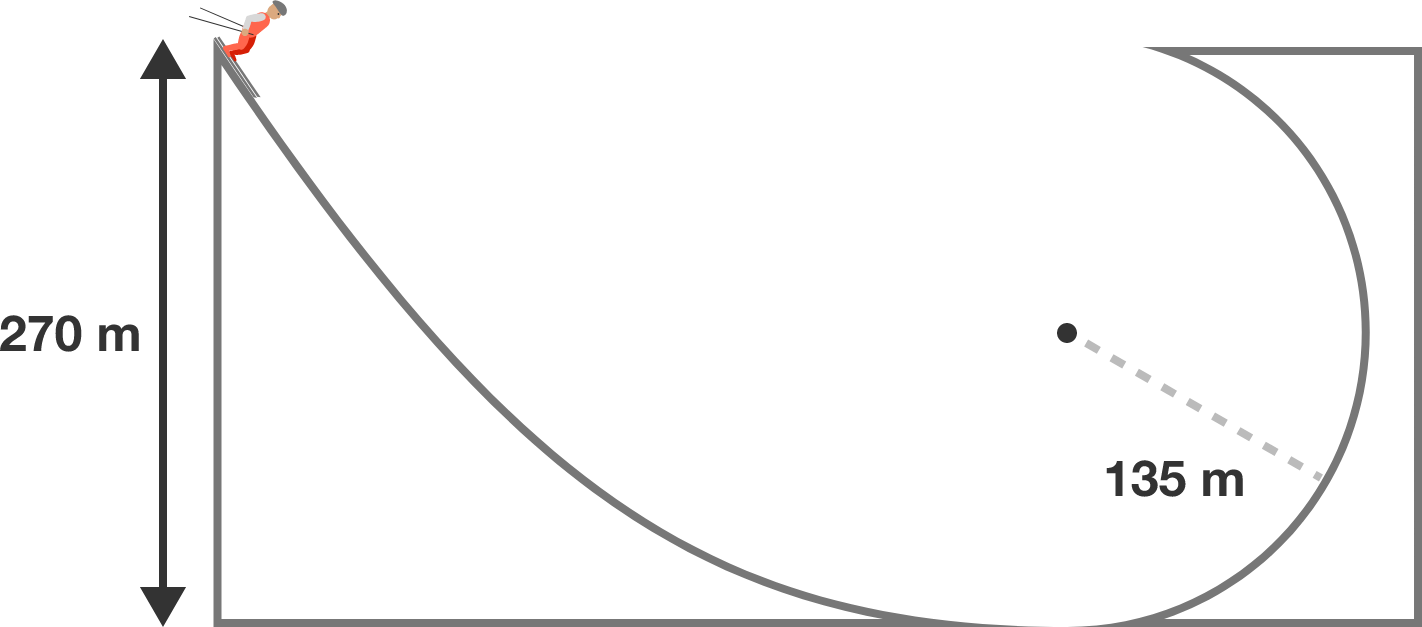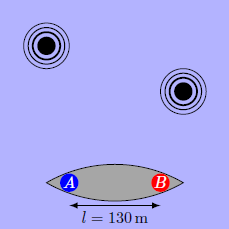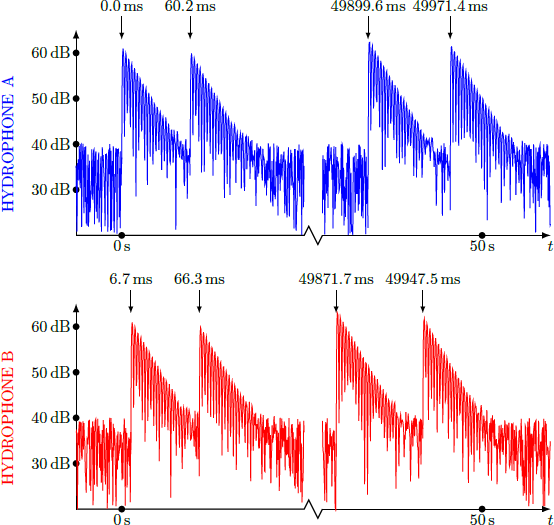# Problems of the Week

Contribute a problem

Given two positive reals $a$ and $b$, the special mean or means are all $\lambda$ such that $\log_{a}{\lambda}=\log_{\lambda}{b}$ or $\log_{b}{\lambda}=\log_{\lambda}{a}.$

Given that at least one special mean of $a$ and $b$ exists, are all special means of $a$ and $b$ between $a$ and $b$ (inclusive)?


Note: This is similar to the way the arithmetic mean of $a$ and $b$ is defined as the $\lambda$ such that $b-\lambda=\lambda-a,$ and the geometric mean of $a$ and $b$ as the $\lambda$ such that $\frac{b}{\lambda}=\frac{\lambda}{a}.$

A skier is about to attempt a daring jump. He starts from the top of a frictionless slope that turns upward into a circular arc, as shown in the figure.

What is the velocity of the skier $($in $\text{m/s})$ at the highest point of his trajectory (after breaking off the slope)?Details and Assumptions:

• Use $g=-10 \text{ m/s}^2.$
• The skier's friends, knowing the monumental stupidity of the skier's stunt, have prepared a net to catch the skier as he falls.

$\large 2017!$

Find the last two non-zero digits in the number above.

Two black holes in a mutually orbiting binary system both have mass $M$ and orbit at radius $R$ to their center of rotation.What is the magnitude of the rate of change of their radius (the rate at which they inspiral due to energy loss to gravitational radiation)?

Assumptions and Details:

• The power radiated in gravitational waves by an inspiraling binary is $P = -\dfrac25 \dfrac{G^4 M^5}{c^5 R^5}.$
• Apart from the expression for gravitational wave radiation, assume Newtonian mechanics applies.A submarine lies in wait and stands still under water at a depth of $d = 200\text{ m}$ to track down an enemy espionage submarine, which is at the same depth. Our submarine has two hydrophones installed at the bow and the stern of the ship at points A and B, which are $l = 130\text{ m}$ apart from each other. The engine of the enemy submarine emits a short and high sound at regular intervals, which is detected by hydrophones. The recorded sound signals for two of these sounds are shown below.

What is the speed of the enemy submarine in $\si[per-mode=symbol]{\meter\per\second}?$

Assume the speed of sound $c = 1500 \text{ m/s}.$Hint: Find out why, in addition to the primary signal, there is another signal that follows the primary signal 60 to 80 milliseconds later.

×